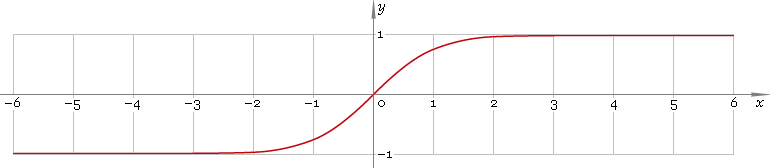The Art of Interface

# tanh or th — hyperbolic tangent function

Category. Mathematics.

Abstract. Hyperbolic tangent: definition, plot, properties and identities.

## 1. Definition

Hyperbolic tangent is defined as

tanhx ≡ (ex − ex) /(ex + ex)

## 2. Plot

Hyperbolic tangent is antisymmetric function defined everywhere on real axis. Its plot is depicted below — fig. 1.Fig. 1. Plot of the hyperbolic tangent function y = tanhx.

Function codomain is limited to the range (−1, 1).

## 3. Identities

Base:

tanh2x + sech2x = 1

By definition:

tanhx ≡ sinhx /coshx ≡ 1 /cothx

Property of antisymmetry:

tanh−x = −tanhx

Half-argument:

tanh(x/2) = (coshx − 1) /sinhx
tanh(x/2) = sinhx /(1 + coshx)
tanhx = 2 tanh(x/2) /[1 + tanh2(x/2)]

Double argument:

tanh(2x) = 2 tanhx /(tanh2x + 1)

Triple argument:

tanh(3x) = (tanh3x + 3 tanhx) /(3 tanh2x + 1)

tanh(4x) = (4 tanh3x + 4 tanhx) /(tanh4x + 6 tanh2x + 1)

Power reduction:

tanh2x = (cosh(2x) − 1) /(cosh(2x) + 1)
tanh3x = (sinh(3x) − 3 sinhx) /(cosh(3x) + 3 coshx)
tanh4x = (cosh(4x) − 4 cosh(2x) + 3) /(cosh(4x) + 4 cosh(2x) + 3)
tanh5x = (sinh(5x) − 5 sinh(3x) + 10 sinhx) /(cosh(5x) + 5 cosh(3x) + 10 coshx)

Sum and difference of arguments:

tanh(x + y) = (tanhx + tanhy) /(1 + tanhx tanhy)
tanh(xy) = (tanhx − tanhy) /(1 − tanhx tanhy)

Product:

tanhx tanhy = [cosh(x + y) − cosh(xy)] /[cosh(x + y) + cosh(xy)]

Sum:

tanhx + tanhy = sinh(x + y) /(coshx coshy)
tanhx − tanhy = sinh(xy) /(coshx coshy)

## 4. Support

Hyperbolic tangent function tanh or th of the real argument is supported by free version of the Librow calculator.

Hyperbolic tangent function tanh or th of the complex argument is supported by professional version of the Librow calculator.

## 5. How to use

To calculate hyperbolic tangent of the number:

``tanh(-1);``

To calculate hyperbolic tangent of the current result:

``tanh(rslt);``

To calculate hyperbolic tangent of the angle x in memory:

``tanh(mem[x]);``